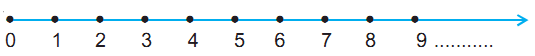# Whole Numbers

Given any natural number, you can add 1 to that number and get the next number i.e. you get its successor. The successor of 16 is 16 + 1 = 17, that of 19 is 19 +1 = 20 and so on. The number 16 comes before 17, we say that the predecessor of 17 is 17 – 1 = 16, the predecessor of 20 is 20 – 1 = 19, and so on. Does 1 have both a successor and a predecessor?

The number 1 has no predecessor in natural numbers.

The natural numbers along with zero form the collection of whole numbers.

When a natural number is subtracted from itself you cannot say what is the left out number. To remove this difficulty, the natural numbers were extended by the number zero (0), to get what is called the system of whole numbers.

Thus, the whole numbers are 0, 1, 2, 3, ....

Like natural numbers, there is no greatest whole number.

### Properties of Whole Numbers

1. Closure property: Whole numbers are closed under addition and also under multiplication.

The sum of any two whole numbers is a whole number. The collection of whole numbers is closed under addition. This property is known as the closure property for addition of whole numbers. The multiplication of two whole numbers is also a whole number. So, the system of whole numbers is closed under multiplication.

The whole numbers are not closed under subtraction or division.

2. Commutativity of addition and multiplication

You can add two whole numbers in any order. Addition is commutative for whole numbers. This property is known as commutativity for addition. Similarly, you can multiply two whole numbers in any order.

Thus, addition and multiplication are commutative for whole numbers.

Subtraction and division are not commutative for whole numbers.

3. Associativity of addition and multiplication

Addition and multiplication, both, are associative for whole numbers.

a + (b + c) = (a + b) + c

4. Distributivity of multiplication over addition

Multiplication is distributive over addition for whole numbers.

Commutativity, associativity and distributivity properties of whole numbers are useful in simplifying calculations and you use them without being aware of them.

5. Identity for addition and multiplication

When you add zero to any whole number, what is the result? It is the same whole number again. Zero is called an identity for addition of whole numbers or additive identity for whole numbers.

Zero has a special role in multiplication too. Any number when multiplied by zero becomes zero.

### Properties of Zero

The number 0 has the following properties:

1. a + 0 = a = 0 + a
2. a – 0 = a but (0 – a) is not defined in whole numbers
3. a × 0 = 0 = 0 × a
4. Division by zero (0) is not defined

### Number Line

Whole numbers can be represented on the number line.Out of any two whole numbers, the number on the right of the other number is the greater number. Whole number on left is the smaller number.